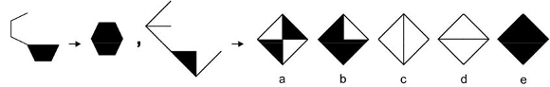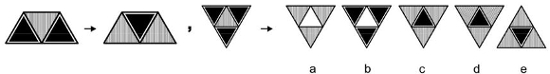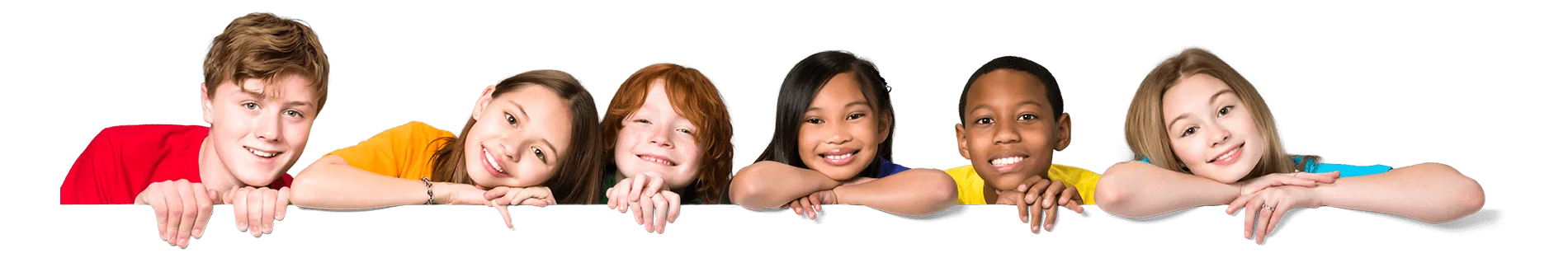Latest Results:

# 11plus Analogy sample papers

Solve this Question

#### On observing the first set, what would be the answer figure in the second set?1. a 2. b 3. c 4. d 5. e

Solve this Question

#### On observing pattern in the first set, what would be the answer figure in the second set?1. a 2. b 3. c 4. d 5. e

#### On observing the first set, what would be the answer figure in the second set?